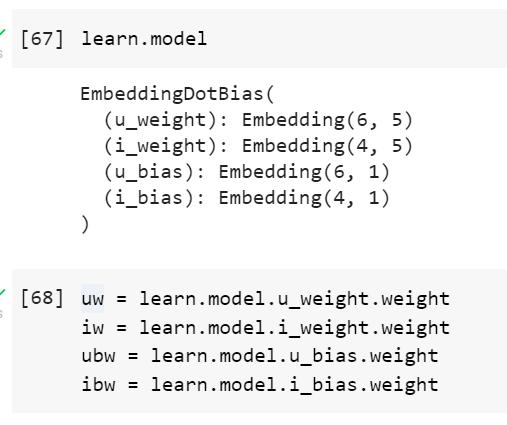# Can't get collab filtering flow

Hi there, great folks! I have a few troubles understanding basic collab example.

Here’s the code I wrote to get better understanding of what’s happening:

``````    ratings = [
[1, 1, 5],
[1, 2, 4],
[1, 3, 1],
[2, 1, 5],
[3, 3, 4],
[4, 2, 1],
[5, 1, 5],
]
ratings = pandas.core.frame.DataFrame(ratings)
print(len(dls.classes)) # n users
print(len(dls.classes)) # n movies

learn = fastai.collab.collab_learner(dls, n_factors=5, y_range=(0, 5.5))
learn.fit_one_cycle(5, 5e-3, wd=0.1)
``````
1. Why do I get 7 users (and sometimes 8 lol) and 4 items, whereas I have only 3 items? I.e. `len(dls.classes))` returns 4 (there’s one #na# item, as I can see).
2. How do I get final recommendations for user 5? I.e. I want to get all the potential ratings, for every movie. Should I do that:
``````user_w = learn.weight()
for i in range(1, 3):
movie_w = learn.weight([i], is_item=True)
print((user_w * movie_w).sum())
``````

Based on what I’ve learned, it should be something like the code above. But that doesn’t work, of course. Even indexing is wrong (because we have 4 movies, not 3, for some reason)

Personally, I’d fake up some more data. (here, m=7 whereas bs=8 )

Oh, yeah, bs is actually 6, fixed.

Yes, I will add more data once I get a better understandingAs for now I’m totally lost in those classes and final weights

For 1, you can

``````print(dls.classes, dls.classes)
``````

To better understand what is considered.For 2, don’t forget that behind the scene there are biases and a sigmoid on top of the sum. The correct formula should be something like (roughly):

``````dot = u_weight * m_weight
res = dot.sum(1) + u_bias + m_bias
res = sigmoid(res) * (y_range - y_range) + y_range
``````
2 Likes
1. `['#na#', 1, 3, 4, 5] ['#na#', 1, 2, 3]`
What is `#na#`? And where is the 2nd user? (It seems that 2nd user is used for validation set, am I right?)

2. Oh thanks! Maybe there’s a shorter way to do that? I saw “predict()” and “get_preds()” methods.

3. Also, are learned `DotProduct.user_factors` analogous to `learn.weight()`?

Also I have DotProduct class from fastai.collab (with sigmoid_range and biases).
But I don’t know how to interpret `movie_factors` or `user_factors` either. I thought I’d just multiply the vectors (since they represent user’s and movie’s latent factors).

I mean, there should be a simple way to recommend concrete movies for concrete users based on that learned model?

Okay, let’s be more concrete``````    def forward(self, x):
users = self.user_factors[x[:, 0]]
movies = self.movie_factors[x[:, 1]]

res = (users * movies).sum(dim=1, keepdims=True)
res += self.user_bias(x[:,0]) + self.movie_bias(x[:,1])

return fastai.layers.sigmoid_range(res, *self.y_range)
``````
``````    model = DotProduct(n_users, n_movies, 4)
learn = flearn.Learner(dls, model, loss_func=flosses.MSELossFlat())
learn.fit_one_cycle(5, 5e-3, wd=0.1)

u_weights = learn.model.user_factors
m_weights = learn.model.movie_factors
u_bias = learn.model.user_bias
m_bias = learn.model.movie_bias
``````

How do I get all best recommended movies (or even better - ranks) for user with `id=5` from here?
I don’t want to multiply whole matrices, just two vectors (user * movie) plus corresponding biases.

Hi,
Multiplication of vectors and matrices should be faster than For loops, so if we want to predict the ratings of user 5 I would go for:

1. copy all model embedding for readability2. Calculate user 5 ratings directly using the model weights:

The predict method didn’t work for me, but I tested using the .get_preds method and it looks fine (user 5 with item 1 has the same rating, which is 2.7484):